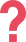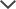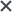The CogAT test pack provided my son with challenging questions, which prepared him to perform well on the test.
Subra
We got the CogAT tests for my daughter. I loved how quickly I was able to access the tests after purchasing the product and how easy it was to use! It familiarized my daughter with the format of the test, allowing her to feel calm for the test.
Margaret
We purchased the NYC G&T pack. We are extremely happy to have purchased the product. It was totally clear and simple and my son felt prepared because we used your pack to prepare. We are very, very happy!
Ketevan

## About the ISEE Math Section

The ISEE Math section assesses mathematical skills learned from the start of a student’s schooling. All of the questions in both of the ISEE Math sections, Quantitative Reasoning and Mathematics Achievements, are associated with the standards of the National Council of Teachers of Mathematics (NCTM). The following NCTM branches are used as the basis for the ISEE Mathematics Achievement section:

• Numbers and Operations
• Algebra
• Geometry
• Measurement
• Data Analysis & Probability
• Problem Solving

Below, you can see an example of an ISEE Math question from an Upper Level ISEE test:

 ISEE Upper level Math Practice Question #1 How many times does 2 appear in the prime factorization of 48? 2 4 5 12 Answer & Explanation ▼ | ▲ The correct answer is 4.

 ISEE Upper level Math Practice Question #2 If (3.75 + 1.25)𝑎 = (11.03 − 1.03)𝑎, what is the value of 𝑎? 0 0.5 5 10 Answer & Explanation ▼ | ▲ The correct answer is 0. In this problem, since 3.75 + 1.25 = 5, the left side of the equation equals 5𝑎. Since 11.03 − 1.03 = 10, the right side of the equation equals 10𝑎. The result is: 5𝑎 = 10𝑎. Assuming that 𝑎 ≠ 0, divide both sides of the equation by 𝑎, and conclude that 5 = 10, which is a false equation. Thus, the only case in which 5𝑎 = 10𝑎 is when 𝑎 = 0, and then 5 × 0 = 10 × 0 = 0, and the correct answer is 0.

This section, unlike the ISEE Quantitative Reasoning section, may actually require students to perform calculations in order to solve problems. Calculators and scratch paper are not permitted on the test. Student are permitted to write in the test booklet.

## ISEE Tips - Math Achievement

Hint: Make sure to read the whole question and examine any related material (such as graphic images) and try to calculate the problem and determine the answer before looking at the answer choices.

Hint: The level of math on the ISEE Mathematics Achievement section may be a higher level of math that you have not yet learned. If you come across a question that you do not understand, guess an answer and continue. If you somewhat understand the question, mark it and come back to it if you have time.

Hint: Many of the wrong answer choices reflect common mistakes. Read each question carefully and more than one time. Remember to CHECK YOUR WORK!

## ISEE Math Achievement Prep

Help your child prepare for the ISEE Math section with TestPrep-Online! We offer ISEE Practice Test packs, which include ISEE Math practice questions. The ISEE Practice Test pack includes realistic practice tests as well as helpful tips, allowing your child to become familiar with the test structure. Help your child start preparing for the ISEE test with TestPrep-Online so that s/he can come to the test with confidence and ready to succeed.

The ISEE and other trademarks are the property of their respective trademark holders. None of the trademark holders are affiliated with TestPrep-Online or this website.

Need HelpNeed Help